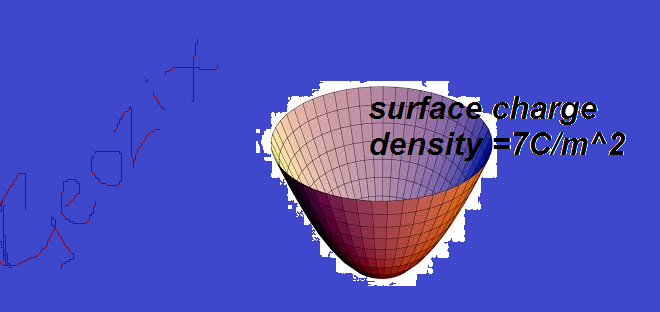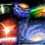There is a paraboloid in space made by revolving $x^2=4z$ about z axis if the structure extends to infinity having a uniform surface charge density. The co- ordinates of the points on the axis in the concave part of the paraboloid where the electric field vanishes is z=infinity but the potential is infinite at two points i.e. at the vertex and at the focus so there should be at least one point where field is 0 where z<1 but electric field decreases uniformly from the vertex to infinite. This is a paradox worth clarifying. could you please share your views with this. $E=\frac{\lambda}{\pi\varepsilon_{0}}\int_{0}^{\infty}\frac{(z-t^{2})*(t^{2}+1)^{0.5}dt}{((z-t^{2})^{2}+4t^{2})^{1.5}}$

$V=\frac{\lambda}{\pi\varepsilon_{0}}\int_{0}^{\infty}\frac{(t^{2}+1)^{0.5}dt}{((z-t^{2})^{2}+4t^{2})^{0.5}}$

at z=0 Vis infinity and at z=1 V=infinite but nowhere between[0,1] is the electric field 0Note by Milun Moghe
7 years, 5 months ago

This discussion board is a place to discuss our Daily Challenges and the math and science related to those challenges. Explanations are more than just a solution — they should explain the steps and thinking strategies that you used to obtain the solution. Comments should further the discussion of math and science.

When posting on Brilliant:

• Use the emojis to react to an explanation, whether you're congratulating a job well done , or just really confused .
• Ask specific questions about the challenge or the steps in somebody's explanation. Well-posed questions can add a lot to the discussion, but posting "I don't understand!" doesn't help anyone.
• Try to contribute something new to the discussion, whether it is an extension, generalization or other idea related to the challenge.

MarkdownAppears as
*italics* or _italics_ italics
**bold** or __bold__ bold
- bulleted- list
• bulleted
• list
1. numbered2. list
1. numbered
2. list
Note: you must add a full line of space before and after lists for them to show up correctly
paragraph 1paragraph 2

paragraph 1

paragraph 2

[example link](https://brilliant.org)example link
> This is a quote
This is a quote
    # I indented these lines
# 4 spaces, and now they show
# up as a code block.

print "hello world"
# I indented these lines
# 4 spaces, and now they show
# up as a code block.

print "hello world"
MathAppears as
Remember to wrap math in $$ ... $$ or $ ... $ to ensure proper formatting.
2 \times 3 $2 \times 3$
2^{34} $2^{34}$
a_{i-1} $a_{i-1}$
\frac{2}{3} $\frac{2}{3}$
\sqrt{2} $\sqrt{2}$
\sum_{i=1}^3 $\sum_{i=1}^3$
\sin \theta $\sin \theta$
\boxed{123} $\boxed{123}$

Sort by:

It is a request to the brilliant staff to look forward to this

- 7 years, 5 months ago

the following expressions are given for a parabola with surface charge density lamda

- 7 years, 5 months ago

The field and potential due to a paraboloid, as described, having surface charge density $\sigma$ is given by:

$E = \displaystyle \frac{\sigma}{ \epsilon_{0}} \int_{0}^{\infty} \frac{\sqrt{1+t} (z-t) dt}{ ((z-t)^2+4t)^{3/2}}$,

$V = \displaystyle \frac{\sigma}{\epsilon_{0}} \int_{0}^{\infty} \frac{\sqrt{1+t} dt}{\sqrt{(z-t)^2 + 4t}}$

- 7 years, 5 months ago

did you take parametric co-ordinates as (t^2,2t) for a parabola $y^2=4x$

- 7 years, 5 months ago

can you please tell me how to post images

- 7 years, 5 months ago

No, $t$ is the vertical height, and $z$ is the height at which electric field and potential is to be found.

- 7 years, 5 months ago

according to my equation t^2 is the vertical height meaning distance of any point on the parabola from the tangent at the vertex and z is the height at which electric and potential to be found

- 7 years, 5 months ago

Even if I substitute $t = x^2$ in my equation , I don't get yours. Btw, couldn't you cancel $\pi$, as the $dA$ term contains $\pi$ and denominator also contains $\pi$ as $4 \pi \epsilon_{0}$.

- 7 years, 5 months ago

Actually i considered only a single parabola of linear charge density considering $y^2 =4x$ for convenience we have to find electric field at x=x

$E=2\int_{0}^{\infty}\frac{(k\lambda(ds))cos\theta}{r^{2}}$

$ds=dx\sqrt{1+(\frac{dy}{dx})^{2}}=d(t^{2})\sqrt{1+(\frac{1}{t})^{2}}=2dt\sqrt{1+t^{2}}$

$r=distance(x,0)\leftrightarrow(t^{2},2t)=\sqrt{(x-t^{2})^{2}+(2t)^{2}}$

$cos\theta=\frac{(x-t^{2})}{r}$

$E=\frac{\lambda}{\pi\varepsilon_{o}}\int_{0}^{\infty}\frac{dt\sqrt{1+t^{2}}(x-t^{2})}{((x-t^{2})^{2}+4t^{2})^{3/2}}$

- 7 years, 5 months ago

Well, you have said that $\lambda$ is surface charge density, and you use it as linear charge density. The fields and potentials are due to whole parabaloid, evaluated by cutting rings.

- 7 years, 5 months ago

Im sorry if i have mentioned lambda as surface charge density. but i just took one parabola with linear charge density lambda . If the above paradox arises with one parabola , then if we take infinitely many parabolas it will form a paraboloid but the paradox for at least one parabola still not solved.

- 7 years, 5 months ago

You can use my expression to evaluate field at focus due to paroboloid. There is no need to cut in parabolas, rather cut in rings, as done by me. Also, your problem was aimed at paraboloid rather than a single parabola, but you ask the field due to single parabola at the focus. Then, what was the need of the story of revolving the parabola and forming a paraboloid and all that?

- 7 years, 5 months ago

I understand your point about integrating rings rather than parabolas. so thats solved . But the single parabola paradox isn't .I thought that if paradox arises with a single parabola it should also be with a paraboloid Thats why i gave a sort of a question . but that isn't true using your method. Well mistakes happen with everyone. Im sorry for that So thats clarified .But now if we only consider a single parabola n the calculate the potential it comes as infinite at the focus as well as the the vertex but nowhere in between is the field zero as it should have been

- 7 years, 5 months ago